Guru

# Which of the following pairs of linear equations are consistent/inconsistent? If consistent, obtain the solution graphically: (i) x + y = 5, 2x + 2y = 10 (ii) x – y = 8, 3x – 3y = 16 (iii) 2x + y – 6 = 0, 4x – 2y – 4 = 0 (iv) 2x – 2y – 2 = 0, 4x – 4y – 5 = 0 Q.4

• 0

What is the different way of solving this important question of  class 10th exercise 3.2 of question no.4 . I am not getting the best way for solving this question and it is also very important question. so please help me for solving this question Which of the following pairs of linear equations are consistent/inconsistent? If consistent, obtain the solution graphically: (i) x + y = 5, 2x + 2y = 10 (ii) x – y = 8, 3x – 3y = 16 (iii) 2x + y – 6 = 0, 4x – 2y – 4 = 0 (iv) 2x – 2y – 2 = 0, 4x – 4y – 5 = 0

Share

1. (i)Given, x + y = 5 and 2x + 2y = 10

(a1/a2) = 1/2

(b1/b2) = 1/2

(c1/c2) = 1/2

Since (a1/a2) = (b1/b2) = (c1/c2)

∴The equations are coincident and they have infinite number of possible solutions.

So, the equations are consistent.

For, x + y = 5 or x = 5 – y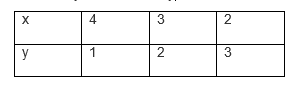For 2x + 2y = 10 or x = (10-2y)/2So, the equations are represented in graphs as follows: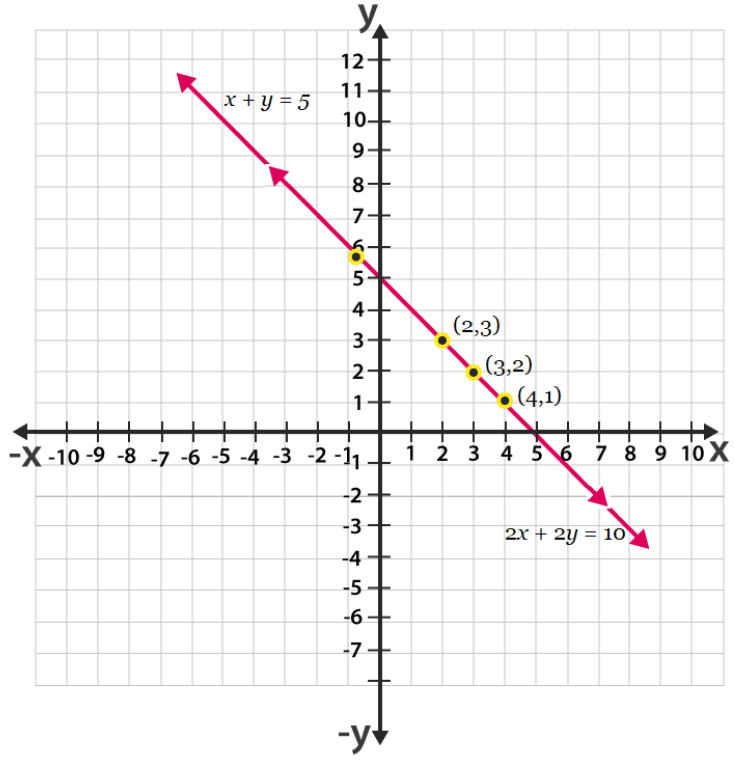From the figure, we can see, that the lines are overlapping each other.

Therefore, the equations have infinite possible solutions.

(ii) Given, x – y = 8 and 3x – 3y = 16

(a1/a2) = 1/3

(b1/b2) = -1/-3 = 1/3

(c1/c2) = 8/16 = 1/2

Since, (a1/a2) = (b1/b2) ≠ (c1/c2)

The equations are parallel to each other and have no solutions. Hence, the pair of linear equations is inconsistent.

(iii) Given, 2x + y – 6 = 0 and 4x – 2y – 4 = 0

(a1/a2) = 2/4 = ½

(b1/b2) = 1/-2

(c1/c2) = -6/-4 = 3/2

Since, (a1/a2) ≠ (b1/b2)

The given linear equations are intersecting each other at one point and have only one solution. Hence, the pair of linear equations is consistent.

Now, for 2x + y – 6 = 0 or y = 6 – 2x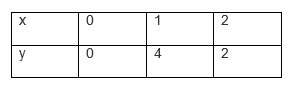And for 4x – 2y – 4 = 0 or y = (4x-4)/2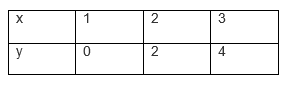So, the equations are represented in graphs as follows: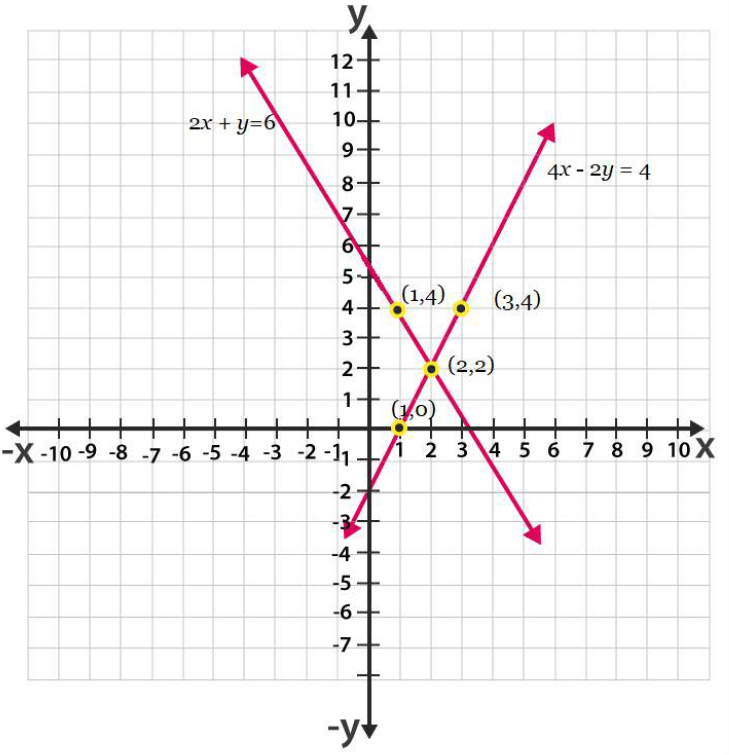From the graph, it can be seen that these lines are intersecting each other at only one point,(2,2).

(iv) Given, 2x – 2y – 2 = 0 and 4x – 4y – 5 = 0

(a1/a2) = 2/4 = ½

(b1/b2) = -2/-4 = 1/2

(c1/c2) = 2/5

Since, a1/a2 = b1/b2 ≠ c1/c2

Thus, these linear equations have parallel and have no possible solutions. Hence, the pair of linear equations are inconsistent.

• 0